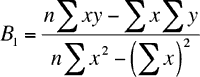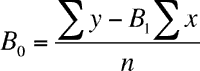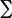Quandaries and Queries

Level: Secondary

Question:
CAN ANYONE HELP ME WITH THIS QUESITON? I AM SO STUCK.
THANKS

I WOULD appreciate it

Hooke's Law asserts that the magnitude of the force required to hold a spring is a linear function of the extension e of the spring. That is,
f = e0 + ke where k and e0 are constants depending only on the spring.
The following data was collected for a spring;

e: 9 , 11 , 12 , 16 , 19
f : 33 , 38 , 43 , 54 , 61

FIND the least square line f= B0 + B1x approximating this data and use it to approximate k.

Hi,

There are a number of equivalent expressions for finding B0 and B1 in the least squares line y = B0 + B1x. In your case the independent variable is e rather than x, the dependent variable is f rather than y and the constants are e0 and k rather than B0 and B1.The formulas I use areandLet's look at your data, changing the variable names from e and f to x and y. You are asked to find k which is the coefficient of e in the equation. After our change of variables to x and y the coefficient of x is B1.

You have n = 5. To use the expressions above I need the sum of all the x's, the sum of the y's, the sum of the x2's and the sum of the x's times the y's. I am going to put your data in a table and calculate these sums.

 x y x2 xy 9 33 92 = 81 933 = 297 11 48 121 528 12 43 144 516 16 54 256 864 19 61 361 1159 67 239 963 3364

The numbers in the bottom row of the table are the sums of the columns. Hencex = 67,y = 239,x2 = 963 andxy = 3364

Now use the expression for B1 above to find the value of k.

Penny

Go to Math Central